Summary

Guide on how to plot directed graphs from igraph objects to display activating and repressive states.

Package

graphsim 1.0.0

# 1 Directed graph plots

## 1.1 Motivations

Here we demonstrate the plotting functions that come built-in with graphsim, an alternative to the plot.igraph provided by the igraph package. Here we provide additional functionality for plotting directed graphs. This draws upon many functions provided by igraph, including layout settings (Csardi and Nepusz 2006).

In particular, graph and network representations in biology often require displaying edge properties (Barabási and Oltvai 2004). Here we have the “state” parameter which can be used to differentiate these, and allow us to represent activating and inhibiting or repressing relationships differently. We use different arrowheads as per convention in molecular biology.

## 1.2 Getting started

To generate these plots, the following packages must be imported.

library("igraph")
library("scales")
library("graphsim")

# 2 Toy example

Here we demonstrate the plot functions on a small toy graph.

## 2.1 Set up simulated graph

First we generate a graph object using the igraph package.

graph_edges <- rbind(c("A", "C"), c("B", "C"), c("C", "D"), c("D", "E"),
c("D", "F"), c("F", "G"), c("F", "I"), c("H", "I"))
graph <- graph.edgelist(graph_edges, directed = TRUE)

## 2.2 Plotting

We next demonstrate the plotting function for directed graph objects. plot_directed with default settings uses the layout.fruchterman.reingold as does built-in plotting function igraph::plot.igraph. This function provides additional functionality to displaying directed graphs in particular.

plot_directed(graph)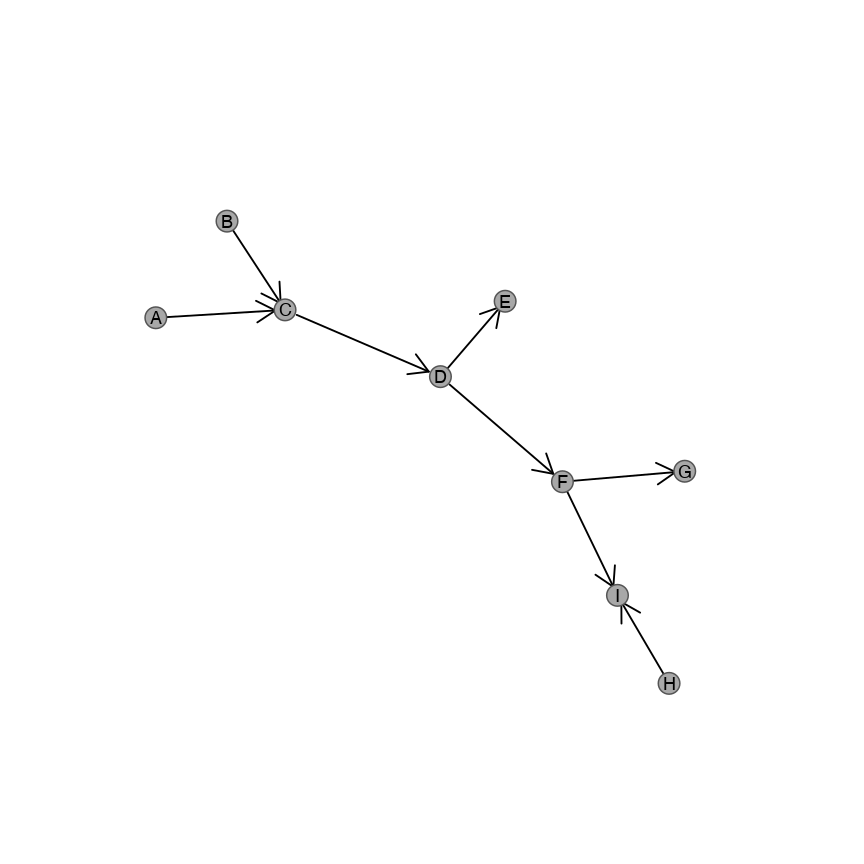Here you can see that the plotting function displays a graph in a similar layout to plot.igraph with different aesthetic parameters. We suggest that you choose the function that suits your needs and datasets. We demonstrate the features available for plot_directed below.

### 2.2.1 Custom aesthetics

We support various aesthetic parameters to control the colour and relative size of nodes and edges.

plot_directed supports customised layouts:

plot_directed(graph, layout = layout.kamada.kawai)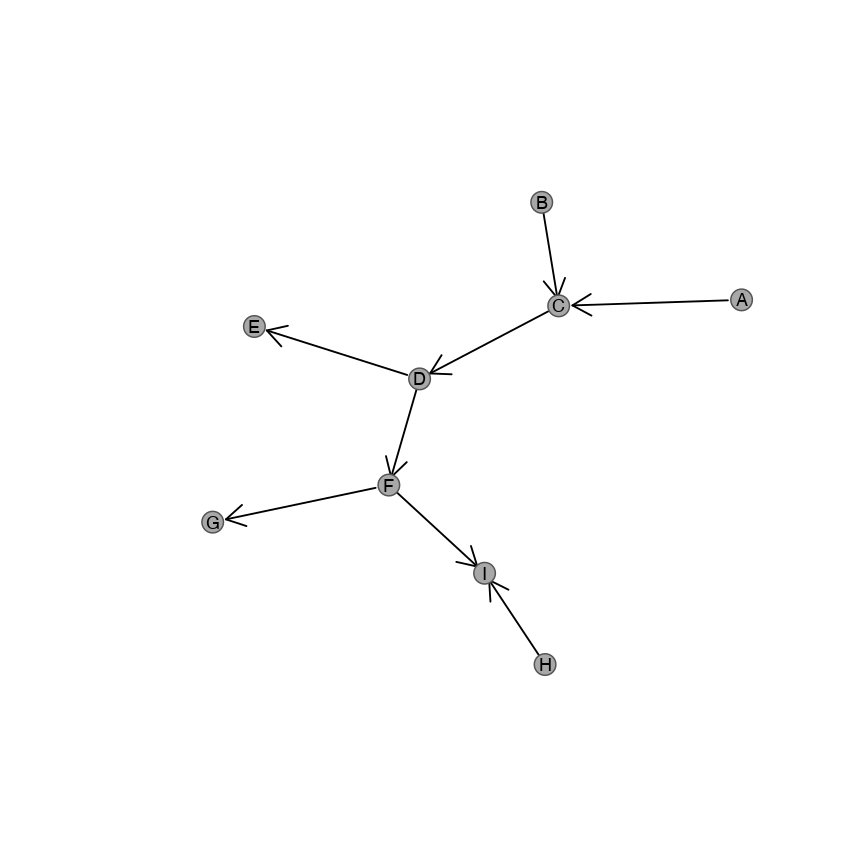In addition, custom colouts are supported:

plot_directed(graph, fill.node = "lightblue", border.node = "royalblue")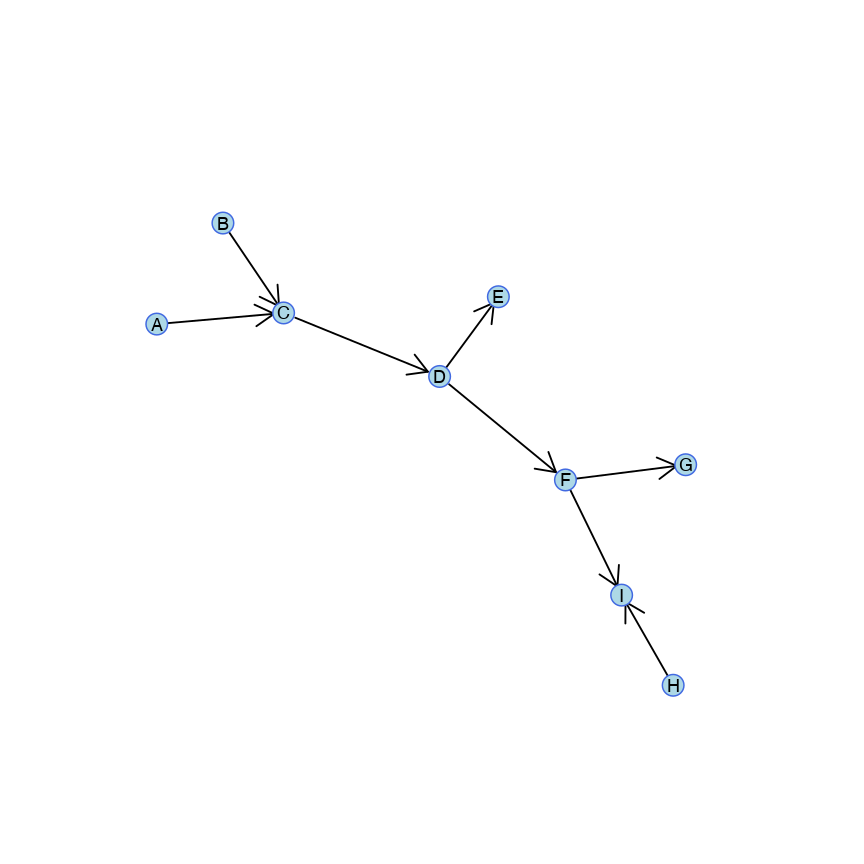#### 2.2.1.1 Vectorisation of aesthetics

Colours may also be entered as a vector for each node in V(graph):

names(V(graph))
##  "A" "C" "B" "D" "E" "F" "G" "I" "H"
colour_vector <- ifelse(names(V(graph)) %in% c("A", "D", "I"), 1, 2)
plot_directed(graph, fill.node = c("lightblue", "grey")[colour_vector], border.node = c("royalblue", "black")[colour_vector])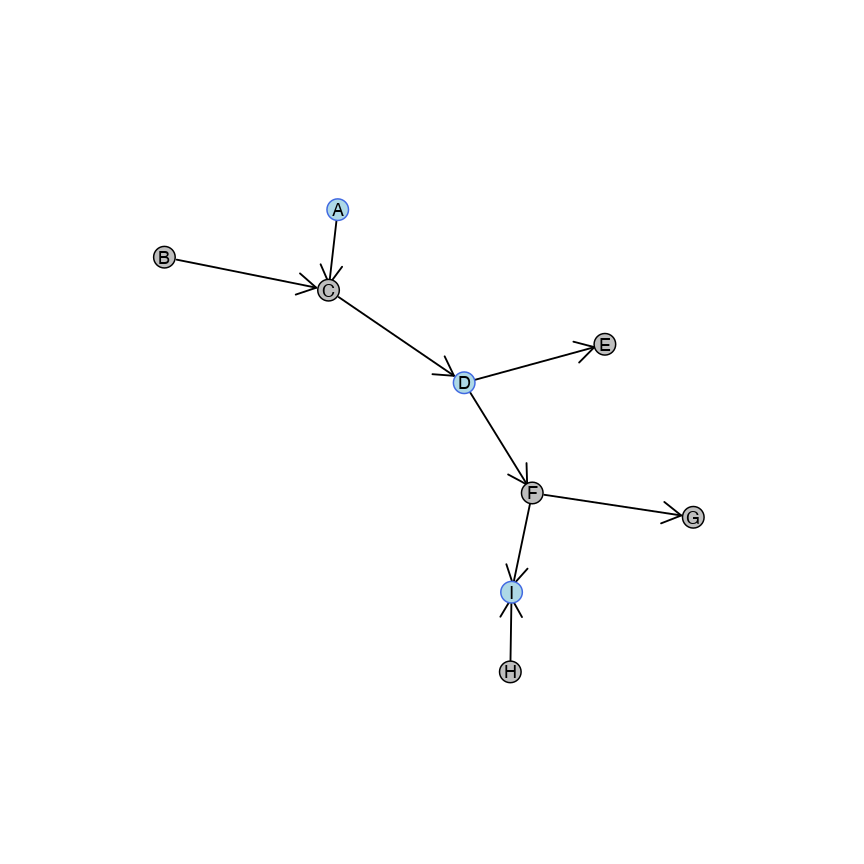This functionality allows highlighting of particular groups based on known properties of the graph. For examples V(graph)$type for bipartite graphs or partitions from Louvain (igraph::cluster_louvain) or Leiden (leiden::leiden) clustering algorithms. ### 2.2.2 Arrow customisation The state parameter controls whether the links are “activating” or “inhibiting”. These can denote activation and repression: foe example, positive and negative regulation of genes or kinase and phosphatase activity of proteins. These may be specified globally as either a character string or numeric: Activating links are displated with any of the following: • “activating” • 1 • 0 plot_directed(graph, state = "activating")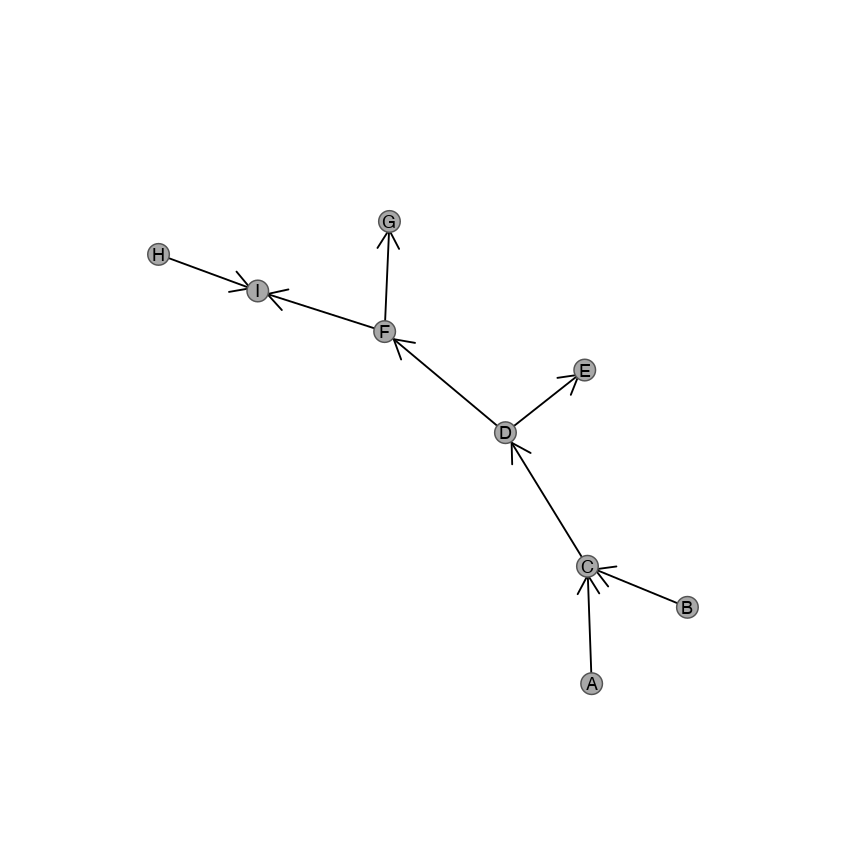Note that activating states can also be specified as follows: plot_directed(graph, state = 1) plot_directed(graph, state = 0) Inhibiting links are displated with any of the following: • “inhibiting” • -1 • 2 plot_directed(graph, state = "inhibiting")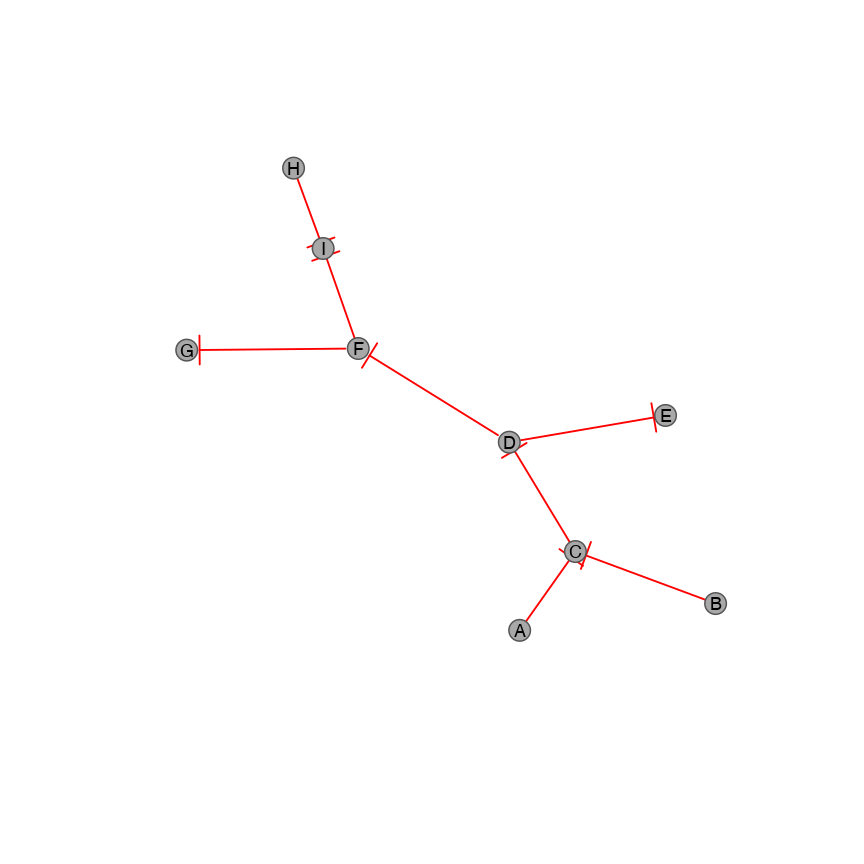Note that inhibiting states can also be specified as follows: plot_directed(graph, state = -1) plot_directed(graph, state = 2) #### 2.2.2.1 Vectorisation of edge properties The state parameter may also be applied as a vector to each edge in E(graph) respectively. E(graph) ## + 8/8 edges from 6213726 (vertex names): ##  A->C B->C C->D D->E D->F F->G F->I H->I plot_directed(graph, state = c(1, 1, -1, -1, 1, -1, 1, -1))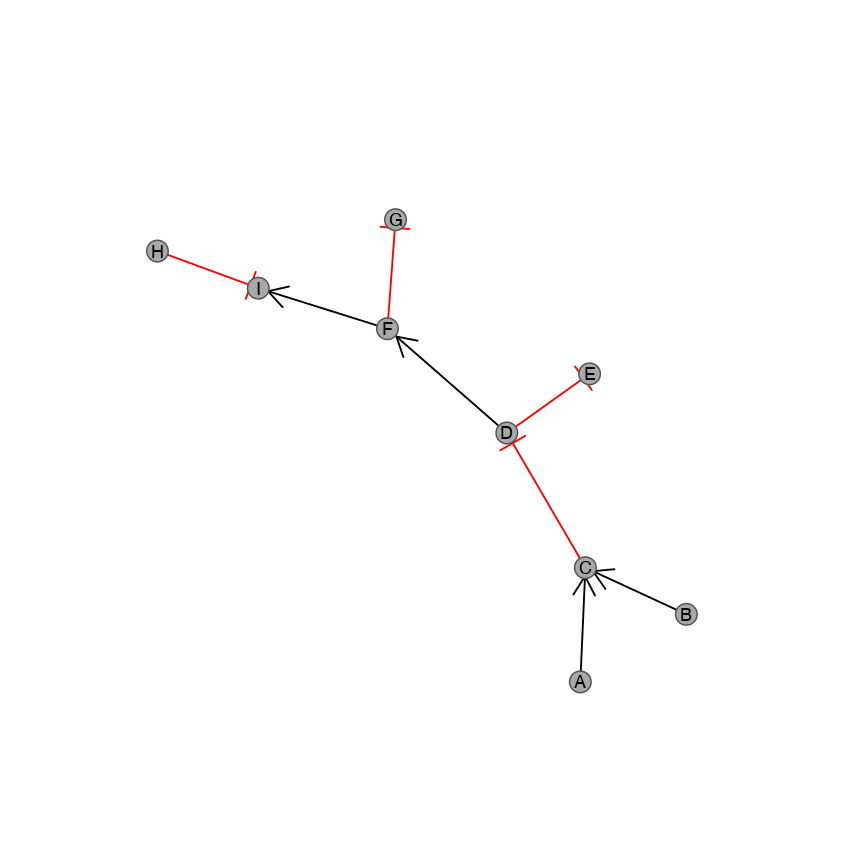Note that by default, inhibiting relationships are highlighted with a different col.arrow value, which can be controlled by the input parameter. edge_properties <- c(1, 1, -1, -1, 1, -1, 1, -1)/2 + 1.5 plot_directed(graph, state = edge_properties, col.arrow = c("darkgreen", "red")[edge_properties])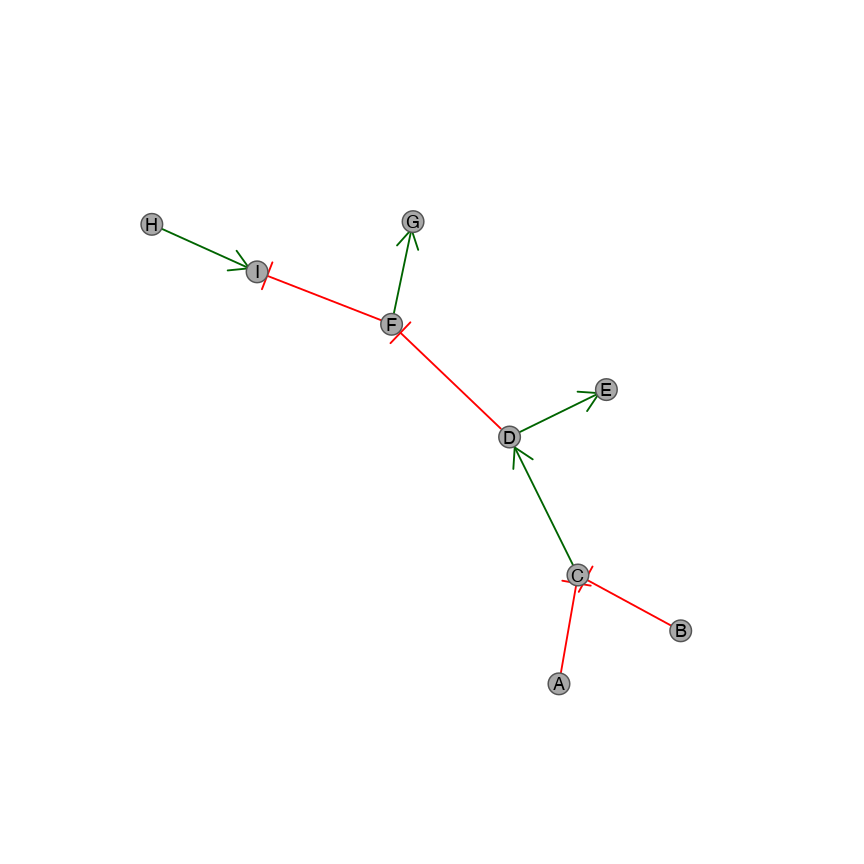edge_properties <- c(1, 1, -1, -1, 1, -1, 1, -1)/2 + 1.5 ggplot_colours <- c("#F8766D", "#CD9600", "#7CAE00", "#00BE67", "#00BFC4", "#00A9FF", "#C77CFF", "#FF61CC") plot_directed(graph, state = edge_properties, col.arrow = ggplot_colours, fill.node = ggplot_colours)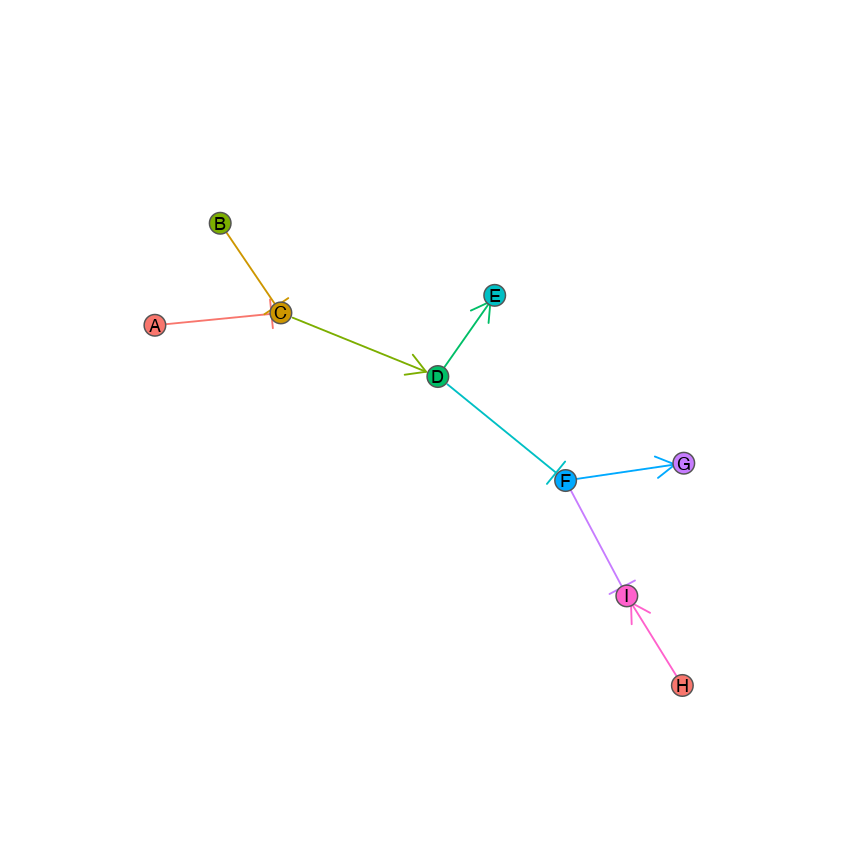# 3 Empirical examples Here we demonstrate using the plotting package to display real biological pathways from the “Reactome” database (Croft et al. 2014). We can import these from the data directory included with this package. These graphs are given for examples and convenience. Any empirical data that consists of a list of directed edges can be imported as an igraph object and handled similarly. Below are some demonstrations. ## 3.1 RAF/MAP kinase cascade Here we plot the RAF/MAP kinase cascade pathway. graph <- identity(RAF_MAP_graph) plot_directed(graph,col.arrow = alpha("#00A9FF", 0.25), fill.node = "lightblue", layout = layout.kamada.kawai)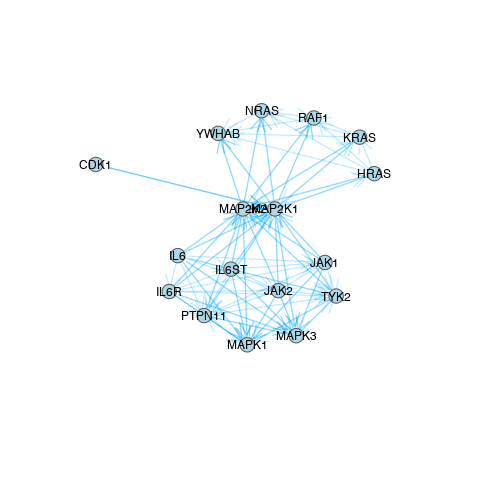## 3.2 Pi3K cascade Here we plot the phosphoinositide-3-kinase (Pi3K) cascade pathway. graph <- identity(Pi3K_graph) plot_directed(graph, col.arrow = alpha("#00A9FF", 0.25), fill.node = "lightblue", layout = layout.kamada.kawai)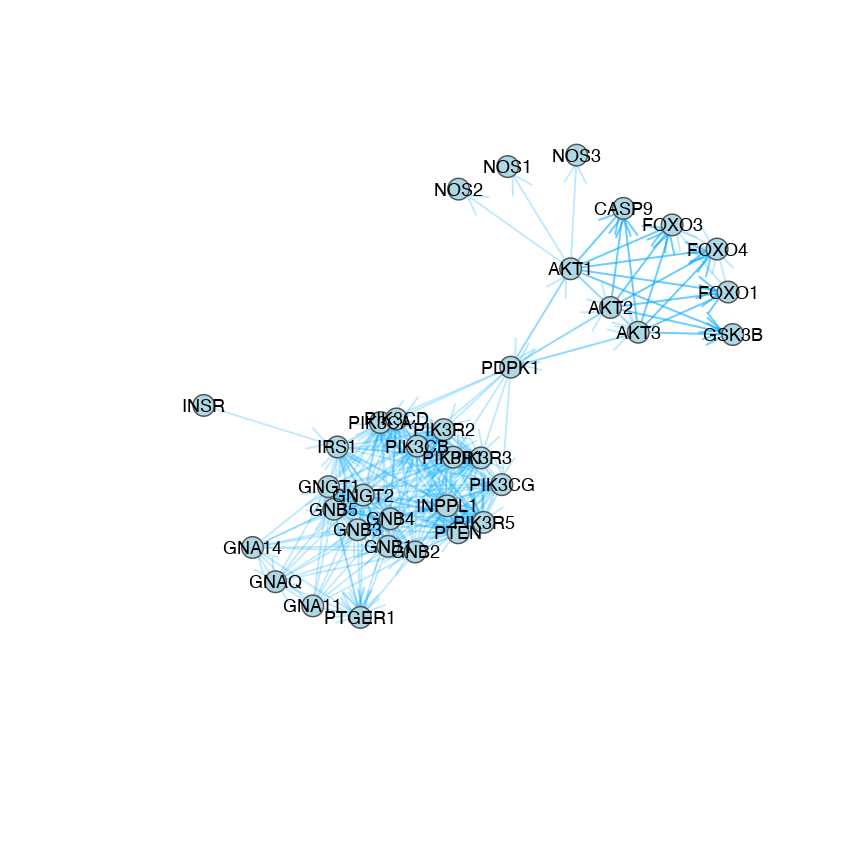## 3.3 TGFβ-Smad pathway Here we plot the TGFβ-Smad pathway with inhibitions known. States are imported as edge attributes from the imported graph. graph <- identity(TGFBeta_Smad_graph) edge_properties <- E(graph)$state
plot_directed(graph, state = edge_properties,
col.arrow = c(alpha("navyblue", 0.25), alpha("red", 0.25))[edge_properties],
fill.node = c("lightblue"),
layout = layout.kamada.kawai)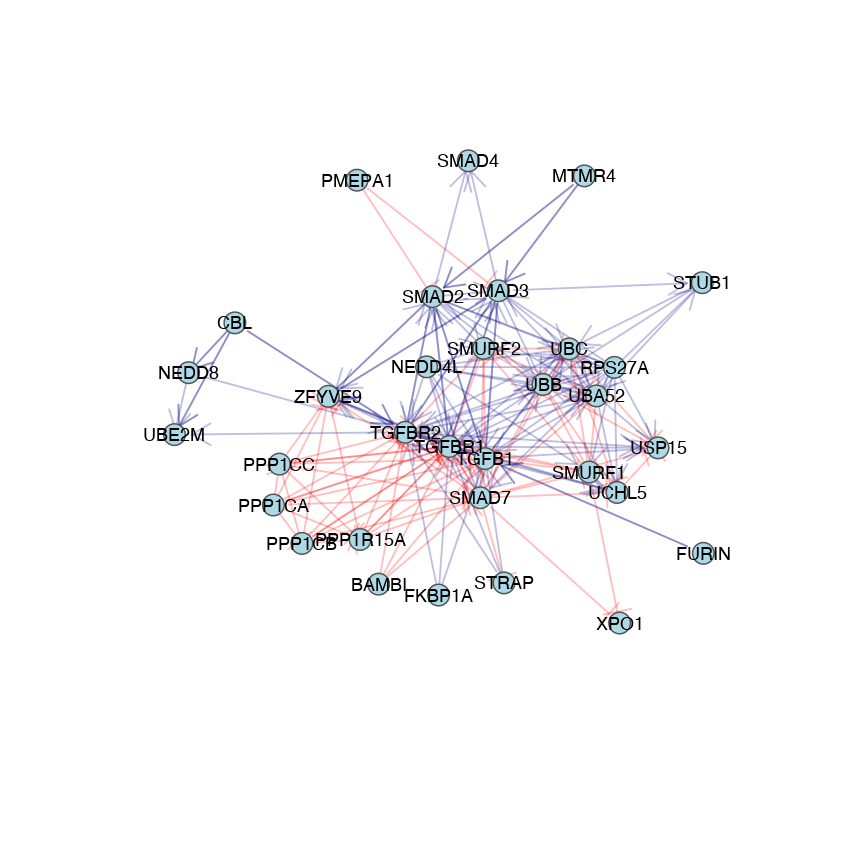# 4 Session info

Here is the output of sessionInfo() on the system on which this document was compiled running pandoc 2.1:

## R version 4.0.2 (2020-06-22)
## Platform: x86_64-apple-darwin17.0 (64-bit)
## Running under: macOS Catalina 10.15.5
##
## Matrix products: default
## BLAS:   /Library/Frameworks/R.framework/Versions/4.0/Resources/lib/libRblas.dylib
## LAPACK: /Library/Frameworks/R.framework/Versions/4.0/Resources/lib/libRlapack.dylib
##
## locale:
##  en_GB.UTF-8/en_GB.UTF-8/en_GB.UTF-8/C/en_GB.UTF-8/en_GB.UTF-8
##
## attached base packages:
##  stats     graphics  grDevices utils     datasets  methods
##  base
##
## other attached packages:
##  graphsim_1.0.1 scales_1.1.1   igraph_1.2.5
##
## loaded via a namespace (and not attached):
##   knitr_1.29         magrittr_1.5       munsell_0.5.0
##   colorspace_1.4-1   lattice_0.20-41    R6_2.4.1
##   rlang_0.4.6        stringr_1.4.0      matrixcalc_1.0-3
##  caTools_1.18.0     tools_4.0.2        grid_4.0.2
##  xfun_0.15          KernSmooth_2.23-17 htmltools_0.5.0
##  gtools_3.8.2       yaml_2.2.1         digest_0.6.25
##  lifecycle_0.2.0    Matrix_1.2-18      farver_2.0.3
##  bitops_1.0-6       prettydoc_0.3.1    evaluate_0.14
##  rmarkdown_2.3      gdata_2.18.0       stringi_1.4.6
##  compiler_4.0.2     gplots_3.0.4       mvtnorm_1.1-1
##  pkgconfig_2.0.3